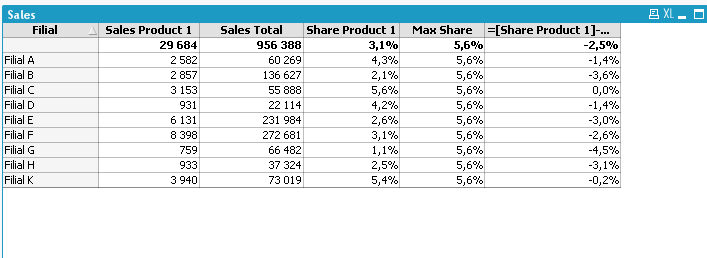# QlikView App Development

Discussion Board for collaboration related to QlikView App Development.

New Contributor III

## Maximum value of strings

Hello.

In the column "Share Product 1", I calculate the share of sales of product 1 from sales total for each filial:

Sum ({<Product = {'Product 1'}>}Amount)/Sum (Amount)I need to calculate the maximum share of product 1 among all the filials and the deviation from this share.

Tags (2)
1 Solution

Accepted Solutions
HighlightedMVP

## Re: Maximum value of strings

Hi Evgen,

Max( Total aggr(Sum ({<Product = {'Product 1'}>}Amount)/Sum (Amount), Filial))

3 Replies
HighlightedMVP

## Re: Maximum value of strings

Hi Evgen,

Max( Total aggr(Sum ({<Product = {'Product 1'}>}Amount)/Sum (Amount), Filial))Partner

## Re: Maximum value of strings

For max share

=max(TOTAL aggr(Sum ({<Product = {'Product 1'}>} Amount)/Sum (Amount), Filial))

Difference

=[Share Product 1]-[Max Share]MVP

## Re: Maximum value of strings

More for the sake of demonstrating Qlik's flexibility in how to calculate various things I present these two alternative expressions that don't use Aggr() to calculate Max Share:

Sum (TOTAL {<Product={'Product 1'},Filial={"=Rank(Sum({<Product={'Product 1'}>} Amount)/Sum(Amount))=1"}>} Amount) / Sum(TOTAL {<Filial={"=Rank(Sum({<Product={'Product 1'}>} Amount)/Sum(Amount))=1"}>} Amount)

or

RangeMax(Top( [Share Product 1],1,NoOfRows()))

Will both calculate the same as:

Max(TOTAL Aggr(Sum ({<Product = {'Product 1'}>} Amount)/Sum (Amount), Filial))